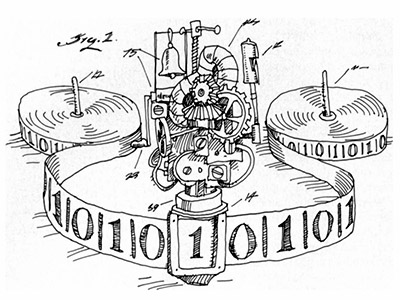ΓραμματείαSecretariat: 2410 684574 | ΦοιτητικάStudents: 2410 684387 g-ds@uth.gr
Select Page

# Computation Theory

Ε801

8

#### Hours/Week - ECTS

4 – 5### Learning Outcomes

The learning objectives of the course are:

• To introduce students to the mathematical infrastructure of computation and specifically to automata theory, the theory of formal languages and grammars, the concepts of algorithm, diagnosability, complexity and computability.
• To develop students’ ability to understand and construct mathematical proofs for calculations and algorithms.

Upon completion of the course the student should be able to:

• categorize abstract machines and construct abstract machines suitable for specific problems
• demonstrate an understanding of the advantages and limitations of analytical techniques in software development
• recognize the importance of complexity classes and calculate the complexity of specific types of algorithms
• demonstrate a deeper and broader understanding of classes of complexity
• prove the basic results of the Theory of Computation

### Indicative Module Content

• INTRODUCTION: Automata, Computability, and Complexity, mathematical concepts and terminology
• REGULAR LANGUAGES: Finite Automata, Arbitrary, Regular Expressions, Non-Regular Languages
• UNCONSUMABLE LANGUAGES: Uncontextualized Grammars, Stack Automata, Non-Uncontextualized Languages,
• THE CHURCH-TURING DOCTRINE: Turing Machines, Variations of Turing Machines, The Definition of Algorithm,
• DIAGNOSTICABILITY: Diagnosable Languages, The Termination Problem
• TIME COMPLEXITY: Measuring Complexity, The P Class, The NP Class
• SPATIAL COMPLEXITY: Savitch’s theorem, The PSPACE class